# Difference between revisions of "Group cohomology of Klein four-group"

View group cohomology of particular groups | View other specific information about Klein four-group

## Classifying space and corresponding chain complex

The classifying space of the Klein four-group is the product space$\mathbb{R}\mathbb{P}^\infty \times \mathbb{R}\mathbb{P}^\infty$, where$\mathbb{R}\mathbb{P}^\infty$ is infinite-dimensional real projective space.

A chain complex that can be used to compute the homology of this space is given as follows:

• The$n^{th}$ chain group is a sum of$n + 1$ copies of$\mathbb{Z}$, indexed by ordered pairs$(i,j)$ where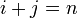$i + j = n$. In other words, the$n^{th}$ chain group is:$\mathbb{Z}_{(0,n)} \oplus \mathbb{Z}_{(1,n-1)} \oplus \dots \mathbb{Z}_{(n,0)}$

• The boundary map is (up to some sign issues that need to be fixed!) given by adding up the following maps:
• The map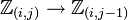$\mathbb{Z}_{(i,j)} \to \mathbb{Z}_{(i,j-1)}$ is multiplication by zero if$j$ is odd and is multiplication by two if$j$ is even.
• The map$\mathbb{Z}_{(i,j)} \to \mathbb{Z}_{(i-1,j)}$ is multiplication by zero if$i$ is odd and multiplication by two if$i$ is even.

## Homology groups

### Over the integers

The homology groups with coefficients in the ring of integers$\mathbb{Z}$ are given as follows:$H_p(\mathbb{Z}/2\mathbb{Z} \oplus \mathbb{Z}/2\mathbb{Z};\mathbb{Z}) = \left\lbrace\begin{array}{rl} (\mathbb{Z}/2\mathbb{Z})^{(p + 3)/2}, & p = 1,3,5,\dots \\ (\mathbb{Z}/2\mathbb{Z})^{p/2}, & p = 2,4,6,\dots \\ \mathbb{Z}, & p = 0 \\\end{array}\right.$

The first few homomology groups are given below:$p$$\! 0$$\! 1$$\! 2$$\! 3$$\! 4$$\! 5$$H_p$$\mathbb{Z}$$\mathbb{Z}/2\mathbb{Z} \oplus \mathbb{Z}/2\mathbb{Z}$$\mathbb{Z}/2\mathbb{Z}$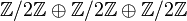$\mathbb{Z}/2\mathbb{Z} \oplus \mathbb{Z}/2\mathbb{Z} \oplus \mathbb{Z}/2\mathbb{Z}$$\mathbb{Z}/2\mathbb{Z} \oplus \mathbb{Z}/2\mathbb{Z}$$\mathbb{Z}/2\mathbb{Z} \oplus \mathbb{Z}/2\mathbb{Z} \oplus \mathbb{Z}/2\mathbb{Z} \oplus \mathbb{Z}/2\mathbb{Z}$

These homology groups can be obtained from the knowledge of the homology groups of cyclic group:Z2 (see group cohomology of cyclic group:Z2) using the Kunneth formula for group homology. They can also be computed explicitly using the chain complex description above.

Here is the computation using the Kunneth formula for group homology: [SHOW MORE]

### Over an abelian group

The homology groups with coefficients in an abelian group$M$ are given as follows: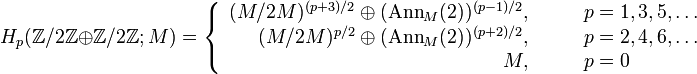$H_p(\mathbb{Z}/2\mathbb{Z} \oplus \mathbb{Z}/2\mathbb{Z};M) = \left\lbrace\begin{array}{rl} (M/2M)^{(p+3)/2} \oplus (\operatorname{Ann}_M(2))^{(p-1)/2}, & \qquad p = 1,3,5,\dots\\ (M/2M)^{p/2} \oplus (\operatorname{Ann}_M(2))^{(p+2)/2}, & \qquad p = 2,4,6,\dots \\ M, & \qquad p = 0 \\\end{array}\right.$

Here,$M/2M$ is the quotient of$M$ by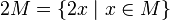$2M = \{ 2x \mid x \in M \}$ and$\operatorname{Ann}_M(2) = \{ x \in M \mid 2x = 0 \}$.

These cohomology groups can be computed in terms of the cohomology groups over integers using the universal coefficients theorem for group homology.

### Important case types for abelian groups

Case on$R$ or$M$ Conclusion about odd-indexed homology groups, i.e.,$H_p, p = 1,3,5,\dots$ Conclusion about even-indexed homology groups, i.e.,$H_p, p = 2,4,6,\dots$$M$ is uniquely 2-divisible, i.e., every element of$M$ has a unique half. This includes the case that$M$ is a field of characteristic not 2. all zero groups all zero groups$M$ is 2-torsion-free, i.e., no nonzero element of$M$ doubles to zero$(M/2M)^{(p+3)/2}$$(M/2M)^{p/2}$$M$ is 2-divisible, but not necessarily uniquely so, e.g.,$M = \mathbb{Q}/\mathbb{Z}$$(\operatorname{Ann}_M(2))^{(p-1)/2}$$(\operatorname{Ann}_M(2))^{(p+2)/2}$$M = \mathbb{Z}/2^n\mathbb{Z}$,$n$ any natural number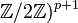$\mathbb{Z}/2\mathbb{Z})^{p+1}$$(\mathbb{Z}/2\mathbb{Z})^{p+1}$$M$ is a finite abelian group isomorphic to$(\mathbb{Z}/2\mathbb{Z})^{r(p + 1)}$ where$r$ is the rank (i.e., minimum number of generators) for the 2-Sylow subgroup of$M$ isomorphic to$(\mathbb{Z}/2\mathbb{Z})^{r(p + 1)}$ where$r$ is the rank (i.e., minimum number of generators) for the 2-Sylow subgroup of$M$$M$ is a finitely generated abelian group all isomorphic to$(\mathbb{Z}/2\mathbb{Z})^{r(p + 1) + s(p + 3)/2}$ where$r$ is the rank for the 2-Sylow subgroup of the torsion part of$M$ and$s$ is the free rank (i.e., the rank as a free abelian group of the torsion-free part) of$M$ all isomorphic to$(\mathbb{Z}/2\mathbb{Z})^{r(p + 1) + sp/2}$ where$r$ is the rank for the 2-Sylow subgroup of$M$

## Cohomology groups and cohomology ring

### Groups over the integers

The cohomology groups with coefficients in the integers are given as below:$H^p(\mathbb{Z}/2\mathbb{Z} \oplus \mathbb{Z}/2\mathbb{Z};\mathbb{Z}) = \left\lbrace \begin{array}{rl} (\mathbb{Z}/2\mathbb{Z})^{(p+1)/2}, & p = 1,3,5,\dots \\ (\mathbb{Z}/2\mathbb{Z})^{(p+2)/2}, & p = 2,4,6,\dots \\ \mathbb{Z}, & p = 0 \\\end{array}\right.$

## Second cohomology groups and extensions

### Schur multiplier

The Schur multiplier, defined as the second cohomology group for trivial group action$H^2(G,\mathbb{C}^\ast)$ and also as the second homology group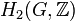$H_2(G,\mathbb{Z})$, is isomorphic to cyclic group:Z2.